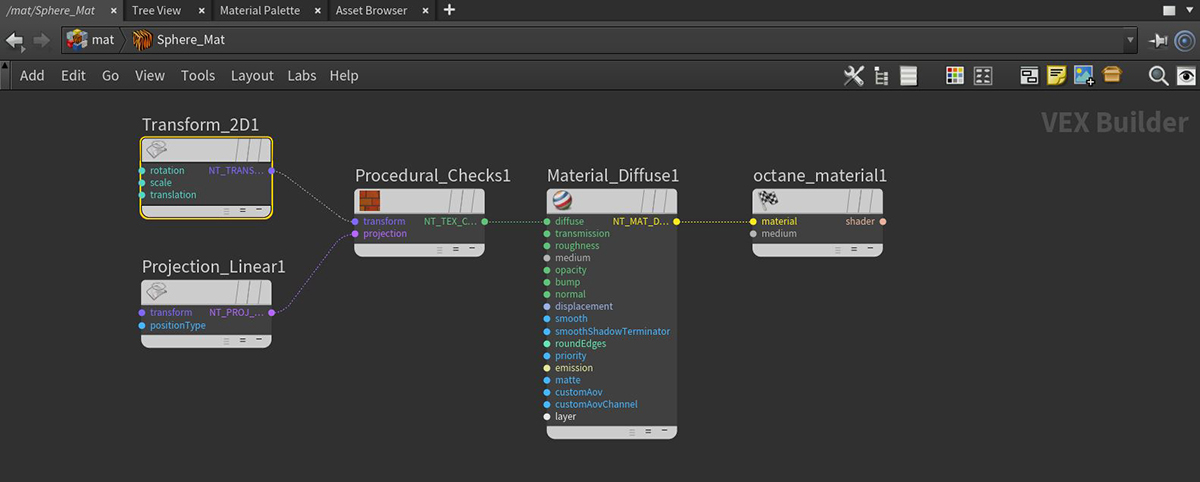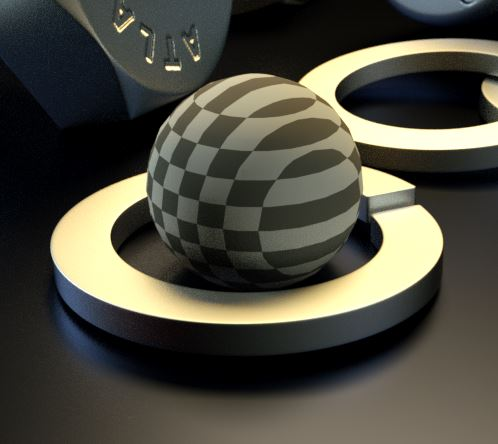# Linear Projection

The Linear projection is also known as planar projection. It differs from Box projection in that the Texture map is projected from a single direction, and not six sides as in Box projections.

If you are using imported Texture maps, then the Linear projection will work as expected with both 2D Transform and 3D Transform nodes.

Note: Linear projections function much like a Box projection if used in a shader network that utilizes a Procedural texture with a 3D Transform node. In order for the Linear projection to produce linear results, use a 2D Transform node when working with Procedural texture maps. This may vary, however, depending on the scene object with which the material is applied.Figure 1: Octane VOP Network exampleFigure 2: Linear projection used with a 2D Transform node on a procedural checks texture